# (8 points) Suppose R is the shaded region in the figure, and f(x, y) is a continuous function on R. Find the limits of integration for the following iterated integral. fx, y) dy dx JA Jc A-...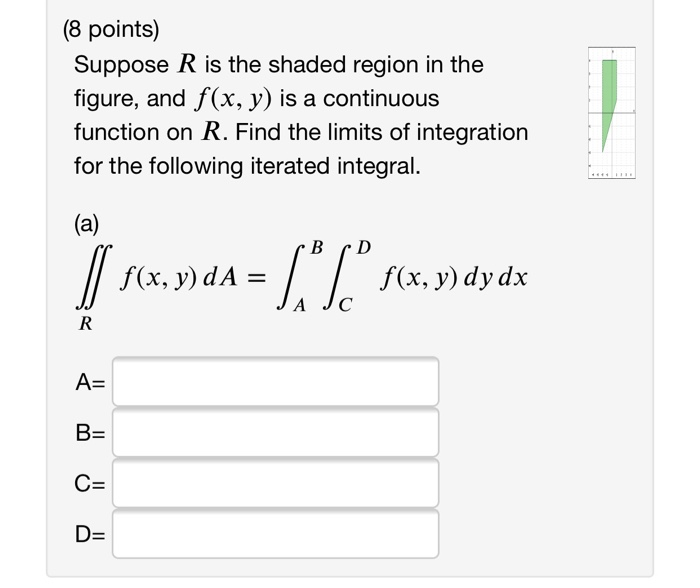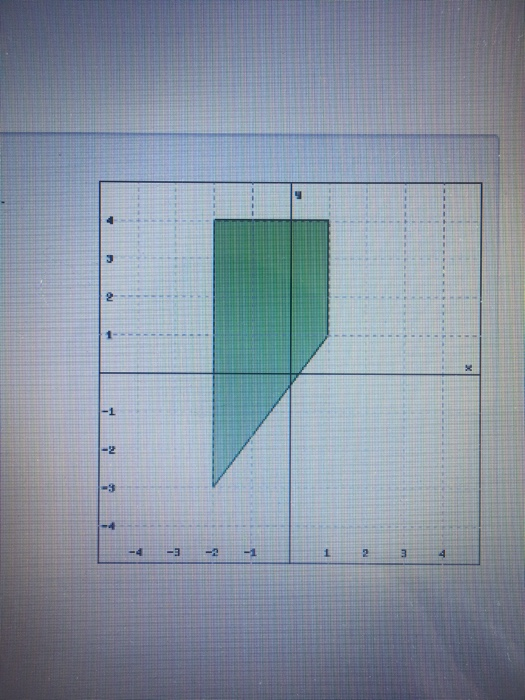(8 points) Suppose R is the shaded region in the figure, and f(x, y) is a continuous function on R. Find the limits of integration for the following iterated integral. fx, y) dy dx JA Jc A- D-
3 2 -1 -2 -3 -4 4 -4 3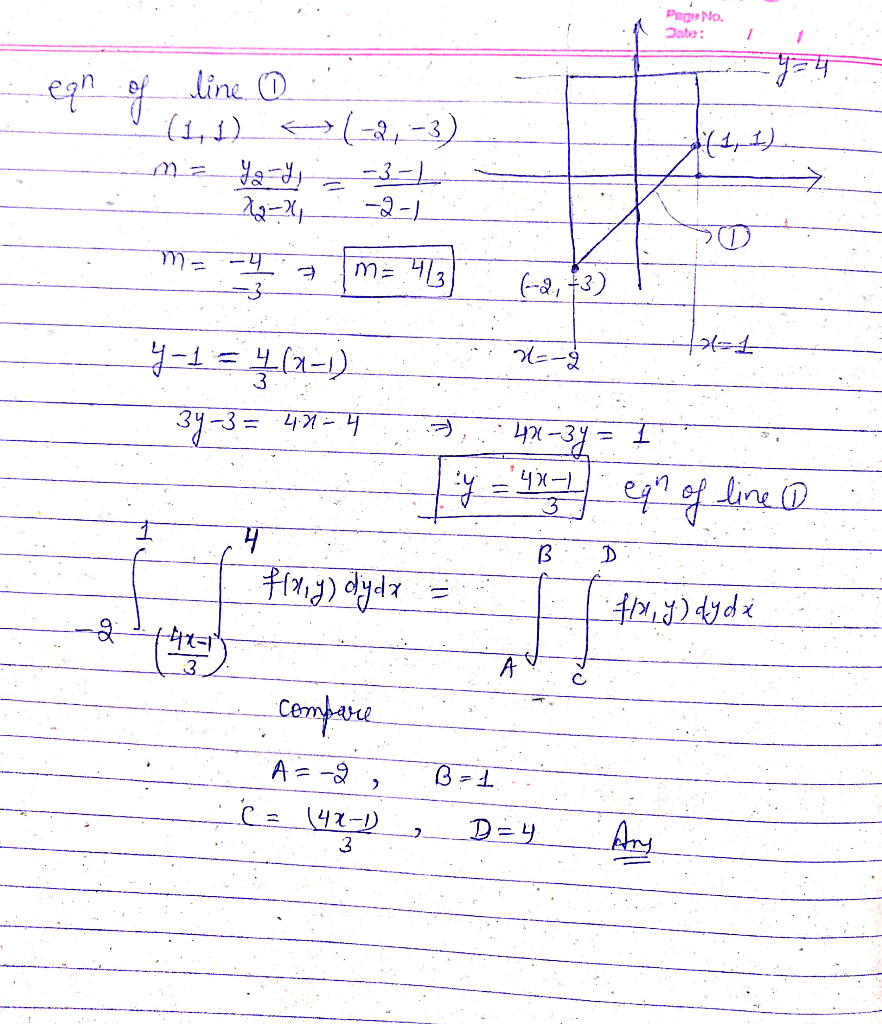##### Add Answer of: (8 points) Suppose R is the shaded region in the figure, and f(x, y) is a continuous function on R. Find the limits of integration for the following iterated integral. fx, y) dy dx JA Jc A-...
Similar Homework Help Questions
• ### Suppose R is the shaded region in the figure, and f(x, y) is a continuous function on R. Find the limits of integration for the following iterated integrals. 3- (a) f(x, y) dA f(x, g) dy dz B- | | f...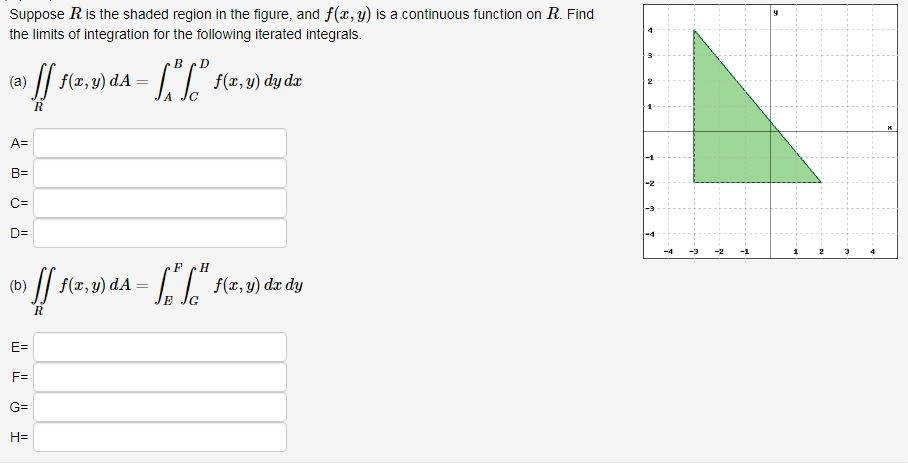Suppose R is the shaded region in the figure, and f(x, y) is a continuous function on R. Find the limits of integration for the following iterated integrals. 3- (a) f(x, y) dA f(x, g) dy dz B- | | f(x,v) dA= f(z, g) dx dy (b) E- F: We were unable to transcribe this image Suppose R is the shaded region in the figure, and f(x, y) is a continuous function on R. Find the limits of integration for...

• ### The figure shows the region of integration for the integral. fx, y, z dy dz dx 0 Jo Rewrite this ...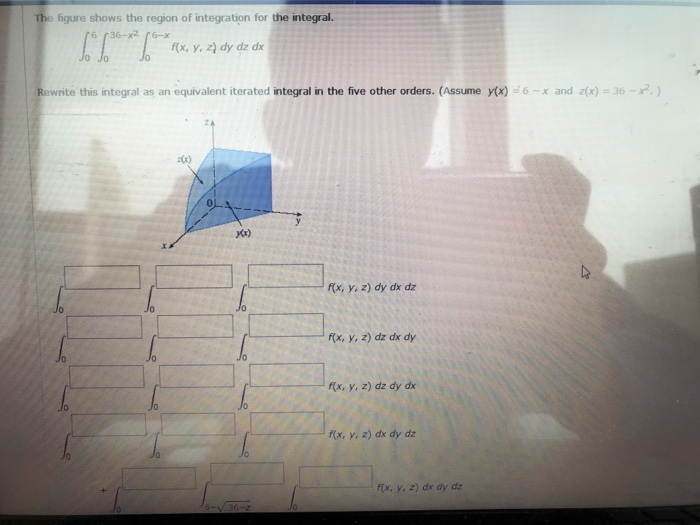The figure shows the region of integration for the integral. fx, y, z dy dz dx 0 Jo Rewrite this integral as an equivalent iterated integral in the five other orders. (Assume yx) 6x and z(x)-36-) x. f(x, y, 2) dy dx dz x, , z) dz dx dy f(x, y, z) dz dy dx f(x, y, z) dx dy dz fx, y, z) dx dy dz J0 Jo Jo f(x, y, z) dz dx dy 0 0 f(x, y,...

• ### 6. [5 marks] Find and classify all stationary points of f(x,y) xy+2xy 7. [6 marks] Sketch the region of integration...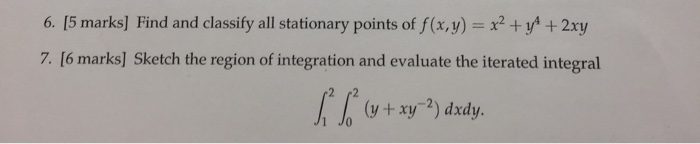6. [5 marks] Find and classify all stationary points of f(x,y) xy+2xy 7. [6 marks] Sketch the region of integration and evaluate the iterated integral 2 r2 JC 10 (y+xy-2) dxdy. 6. [5 marks] Find and classify all stationary points of f(x,y) xy+2xy 7. [6 marks] Sketch the region of integration and evaluate the iterated integral 2 r2 JC 10 (y+xy-2) dxdy.

• ### The figure shows the region of integration for the given intsgral. Rewrite the integral as an equ...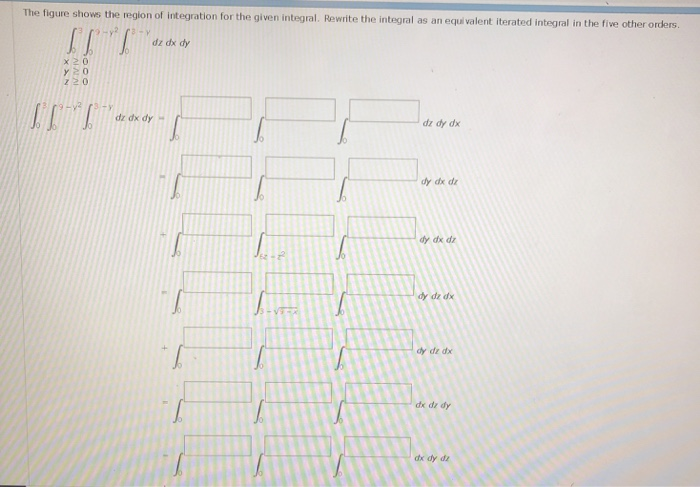The figure shows the region of integration for the given intsgral. Rewrite the integral as an equ valent iterated integral in the five other orders z20 dz dy dx dy dx dz dy dx dz dy de dx dx dz dy dx dy dz dx dy dz z. 2 3 z-3-y 0 The figure shows the region of integration for the given intsgral. Rewrite the integral as an equ valent iterated integral in the five other orders z20 dz dy...

• ### Fx 0. Show that =-- dx Fy dy 8. Suppose y is a function of z, F(x, y) = 0, and F,メO. Show that d...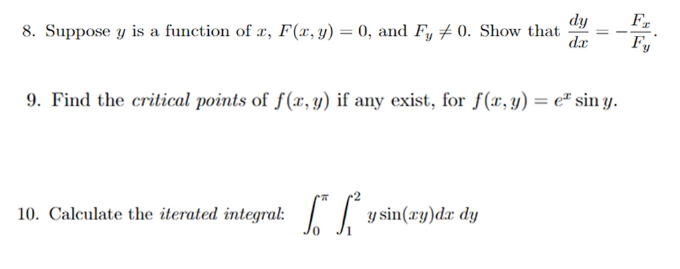Fx 0. Show that =-- dx Fy dy 8. Suppose y is a function of z, F(x, y) = 0, and F,メO. Show that dr--Fr 9. Fid the critical points of f(z, y) if any exist, for (a, y) = ex sin y 10. Calculate the iterated integral: ysin(zy)d dy Fx 0. Show that =-- dx Fy dy 8. Suppose y is a function of z, F(x, y) = 0, and F,メO. Show that dr--Fr 9. Fid the critical points...

• ### Problem 5 [10 points] Set up integrals for both orders of integration. Use the more convenient order to evaluate the integral over the plane region R: A R region bounded by y 0, y x, x 4 R 1+x2 a...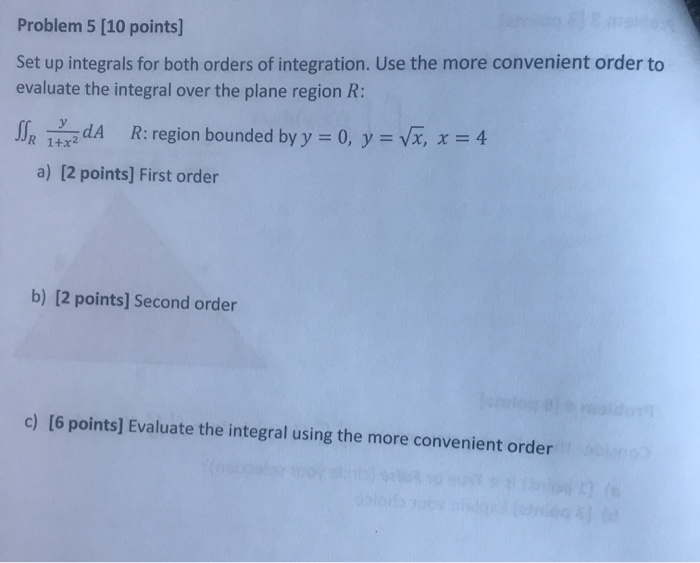Problem 5 [10 points] Set up integrals for both orders of integration. Use the more convenient order to evaluate the integral over the plane region R: A R region bounded by y 0, y x, x 4 R 1+x2 a) [2 points] First order b) [2 points] Second order c) [6 points] Evaluate the integral using the more convenient order Problem 5 [10 points] Set up integrals for both orders of integration. Use the more convenient order to evaluate the...

• ### 6. -1.25 points My Notes Evaluate (y 3 sin x) dx + (z2 +7 cos y) dy x3 dz COS JC where C is the c...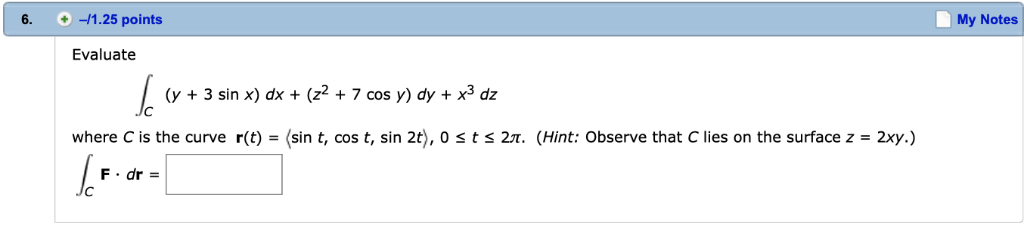6. -1.25 points My Notes Evaluate (y 3 sin x) dx + (z2 +7 cos y) dy x3 dz COS JC where C is the curve r(t) - (sin t, cos t, sin 2t), 0 s t s 27. (Hint: Observe that C lies on the surface z - 2xy.) F dr- 6. -1.25 points My Notes Evaluate (y 3 sin x) dx + (z2 +7 cos y) dy x3 dz COS JC where C is the curve r(t) -...

• ### R+9 tan θ Find the area of the shaded region in the accompanying 2 figure. Is the graph of r = 9 ...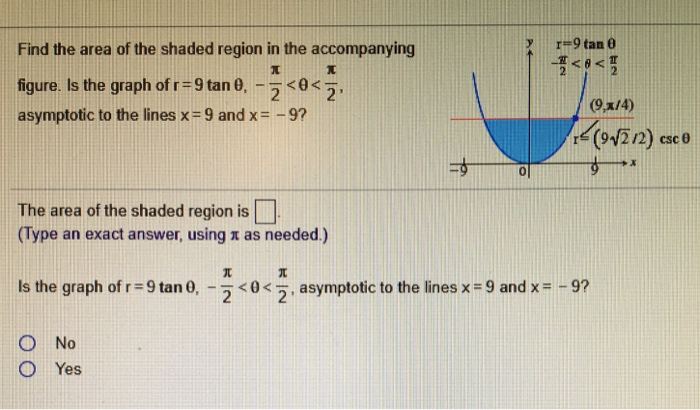r+9 tan θ Find the area of the shaded region in the accompanying 2 figure. Is the graph of r = 9 tan 0,-2 <0 < 7: asymptotic to the lines x = 9 and x =-9? 9x/4) -(912 /2) csc θ ol The area of the shaded region is (Type an exact answer, using π as needed.) is the graph of r = 9 tan 0,-2 < 0 <乏, asymptotic to the lines x-9 and x =-9? ○No O...

• ### Answer the following questions: a) b) c) Suppose fx,v) and g(x,y) are functions in the plane, and fx,y2gx, for all x and y in a region R. What geometric quantity does fx,y-g(x,y)dA represent? Suppo...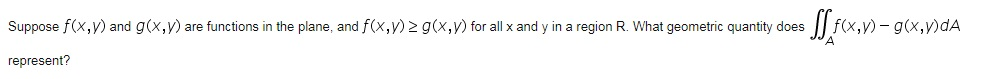Answer the following questions: a) b) c) Suppose fx,v) and g(x,y) are functions in the plane, and fx,y2gx, for all x and y in a region R. What geometric quantity does fx,y-g(x,y)dA represent? Suppose a region A is easily described as the region between one curve on the left and another curve on the right. When computing thed would you integrate over X first, or over V first? If R is a region in the plane, what geometric quantity does...

• ### (a) Evaluate the double integral 4. (sin cos y) dy dr. Hint: You may need the formula for integration by parts (b) Show...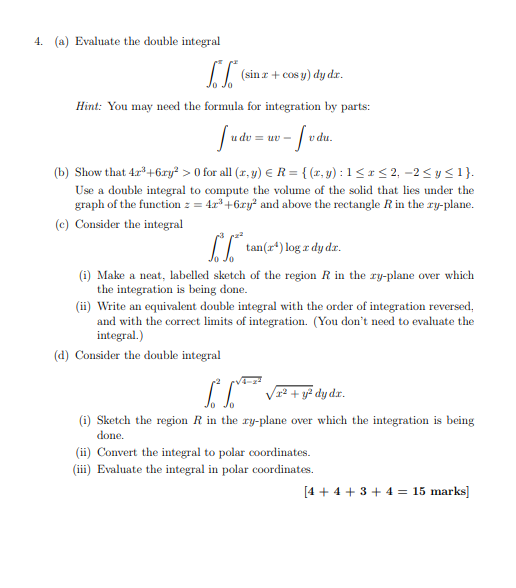(a) Evaluate the double integral 4. (sin cos y) dy dr. Hint: You may need the formula for integration by parts (b) Show that 4r+6ry>0 for all (r,y) ER-(x,y): 1S2,-2Sysi) Use a double integral to compute the volume of the solid that lies under the graph of the function 4+6ry and above the rectangle R in the ry-plane. e) Consider the integral tan(r) log a dyd. (i) Make a neat, labelled sketch of the region R in the ry-plane over...

Need Online Homework Help?